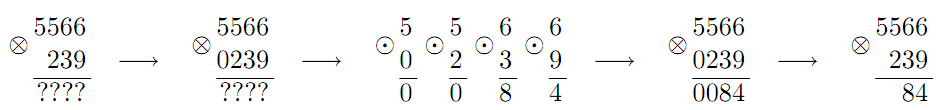Имя: Пароль: Зарегистрироваться Восстановить пароль
25/03/2023 05:37:56

#Рабочее место участника

##Задачи

1869. Binary Operation

Ограничения: время – 2s/4s, память – 256MiB Ввод: input.txt или стандартный ввод Вывод: output.txt или стандартный выводПослать решение Blockly Посылки Темы Где Обсудить (0)

Consider a binary operation ⊙ defined on digits 0 to 9, ⊙:\ {0,1,…,9}\ ×\ {0,1,…,9}\ →\ {0,1,…,9}, such that 0\ ⊙\ 0\ =\ 0.
A binary operation ⊗ is a generalization of ⊙ to the set of non-negative integers, ⊗:\ Z_{0+}\ ×\ Z_{0+}\ →\ Z_{0+}. The result of a\ ⊗\ b is defined in the following way: if one of the numbers a and b has fewer digits than the other in decimal notation, then append leading zeroes to it, so that the numbers are of the same length; then apply the operation ⊙ digit-wise to the corresponding digits of a and b.Example. If a\ ⊙\ b\ =\ "ab"\ mod\ 10, then 5566\ ⊗\ 239\ =\ 84.
Let us define ⊗ to be left-associative, that is, a\ ⊗\ b\ ⊗\ c is to be interpreted as (a\ ⊗\ b)\ ⊗\ c.
Given a binary operation ⊙ and two non-negative integers a and b, calculate the value of a\ ⊗\ (a+1)\ ⊗\ …\ ⊗\ (b-1)\ ⊗\ b.
The first ten lines of the input file contain the description of the binary operation ⊙. The i-th line of the input file contains a space-separated list of ten digits –- the j-th digit in this list is equal to (i-1)\ ⊙\ (j-1).
The first digit in the first line is always 0.
The eleventh line of the input file contains two non-negative integers a and b (0\ ≤\ a\ ≤\ b\ ≤\ 10^{18}).
Output a single number –- the value of a\ ⊗\ (a+1)\ ⊗\ …\ ⊗\ (b-1)\ ⊗\ b without extra leading zeroes.

Sample Input

0 1 2 3 4 5 6 7 8 9
1 2 3 4 5 6 7 8 9 0
2 3 4 5 6 7 8 9 0 1
3 4 5 6 7 8 9 0 1 2
4 5 6 7 8 9 0 1 2 3
5 6 7 8 9 0 1 2 3 4
6 7 8 9 0 1 2 3 4 5
7 8 9 0 1 2 3 4 5 6
8 9 0 1 2 3 4 5 6 7
9 0 1 2 3 4 5 6 7 8
0 10


Sample Output

15

Source: ACM ICPC NEERC, 2010